﻿ Application of Grey Numbers to Assessment of the Understanding the Graphical Representation of the DerivativePublications are Open
Access in this journal
Article Versions
Export Article
• Normal Style
• MLA Style
• APA Style
• Chicago Style
Research Article
Open Access Peer-reviewed

### Application of Grey Numbers to Assessment of the Understanding the Graphical Representation of the Derivative

Michael. Gr. Voskoglou
American Journal of Educational Research. 2017, 5(11), 1167-1171. DOI: 10.12691/education-5-11-9
Published online: December 06, 2017

### Abstract

Grey numbers, which are defined with the help of the real intervals, are very useful in the everyday life for handling approximate data. In the present paper grey numbers are used as a tool for assessing, with linguistic expressions, the student understanding of the graphical representation of the derivative. Although the proposed new assessment method is proved to be equivalent with an analogous method using Triangular Fuzzy Numbers developed in earlier works, the required computational burden is significantly reduced. A classroom application is also presented illustrating our results.

### 1. Introduction

Mathematical education researchers report that, although student routine performance on differentiation items is usually adequate, most of them have little intuitive or conceptual understanding of the derivative concept 1, 2, 3, 4, 5, 6. Many calculus students are, for example, proficient at differentiating a function and finding its critical values. However, they face difficulties to conceptualize these actions and to work with them if they are not presented in equation form.

It is recalled that the formal definition of the derivative f΄(a) of a function y = f(x) at a point a of its domain is given by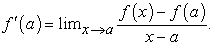(1)

However, the concept of the limit is difficult to be understood by students. For example, in calculating the limit appearing in definition (1), while we initially accept that x tends to α with values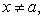to calculate it we finally set x = a. Although this can be easily justified by the continuity of y = f(x) at α, it is frequently confusing the novices. It is characteristic that 150 whole years were required from the time that (1642-1727) and Leibniz (1646-1716) initiated the Differential Calculus until the complete understanding of the concept of the limit.

It seems that many students perform poorly due to their weakness to deal successfully with information about abstract concepts (e.g. functions, limits etc.) given in symbolic form, but also because they luck of the necessary cognitive schemas that could allow them to organize their knowledge on those matters 7. Consequently, the use by the instructor of various representations of the concept of the derivative is recommended as well as the student orientation to analogous activities 8, 9, 10, 11. Such representations involve for example the rate of change of the function f(x) with respect to x, the physical nature of the derivative connected to the speed and to the acceleration at a moment of time of a moving object under the action of a steady force and its geometric representation as the slope of the tangent at the point (a,f(a)) of the graph of y = f(x).

In the present paper grey numbers (GNs) will be used as tools for assessing student difficulties for the graphical understanding of the derivative. The rest of the paper is formulated as follows: In Section 2 we discuss the assessment methods that we have used in earlier works. In Section 3 we introduce the necessary for the purposes of the present paper background from the theory of GNs. In Section 4 we develop the new assessment method, while in Section 5 we present a classroom experiment on assessing student understanding of the graphical representation of the derivative. The paper closes with our conclusion and hints for further research.

### 2. Assesment Methods

The assessment of a system’s effectiveness, i.e. of the degree of attainment of its targets) with respect to an activity performed within the system (e.g. problem-solving, decision making, learning, etc) is a very important task, which enables the correction of the system’s weaknesses resulting to the improvement of its general performance. The traditional assessment methods that are usually applied in practice are based on principles of the traditional bivalent logic (yes-no). However, assessment situations appear frequently in real life characterized by a degree of uncertainty and/or ambiguity. For example, a teacher is frequently not sure for a particular numerical grade characterizing a student’s performance. In such cases fuzzy logic, due to its property of characterizing the ambiguous/uncertain situations with multiple values, offers rich resources for the assessment purposes.

The traditional method for assessing a group’s mean performance is the calculation of the mean value of its members’ numerical scores. However, frequently in practice the individual performance is evaluated not by numerical scores, but by qualitative characterizations (grades), like excellent, very good, good, fair, unsatisfactory, etc. In such cases, in which the calculation of the mean value is not possible, one could use the also traditional method of calculating the Grade Point Average (GPA) index (e.g. see 12, p. 125) for assessing the group’s performance. However GPA actually measures not the mean, but the group’s quality performance, by assigning greater coefficients to the higher scores.

In earlier works we have used the measurement of a fuzzy system’s uncertainty as a tool for assessing a group’s mean performance (e.g. see 12, Chapter 5). Nevertheless, this method, apart of requiring laborious calculations, can be used to compare the mean performance of two different groups only under the assumption that the existing uncertainty in the two groups before the relative activity in which they are participating is the same, a condition which is not always true.

More recently we have also used fuzzy numbers as tools for evaluating a group’s mean performance (e.g. see 12, Chapter 7, 13, etc.), which appears to be a more general and accurate method than the measurement of the uncertainty. The method that will be developed in this paper by using GNs as assessment tools is actually equivalent to the method using the special form of the triangular fuzzy numbers (TFNS). (see Remark of Section 3), but it reduces significantly the required computational burden.

### 3. Grey Numbers

Frequently in the everyday life, as well as in many applications of science and engineering including medicine diagnostics, psychology, sociology, control systems, economy price indices, opinion polls, etc., the data can not be easily determined precisely and in practice estimates of them are used. Apart from fuzzy logic, another effective tool for handling the approximate data is the use of the GNs, which are introduced with the help of the real intervals.

A GN is an indeterminate number whose probable range is known, but which has unknown position within its boundaries. Therefore, if R: denotes the set of real numbers, a GN, say A, can be expressed mathematically by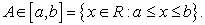Compared with the interval [a, b] the GN A enriches its uncertainty representation with the whitenization function, defining a degree of greyness for each x in [a, b]. When a=b, then A is called a white number and if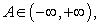then A is called a black number. For general facts on GNs we refer to the book 14.

From the definition of the GNs it becomes evident that the well known arithmetic of the real intervals 15 can be used to define the basic arithmetic operations among the GNs. More explicitly, if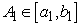and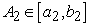are given GNs, then we define:

Addition by: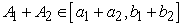Subtraction by: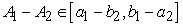Multiplication by: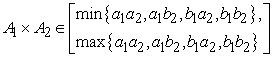Division by: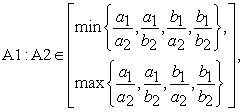where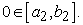Scalar multiplication by: kA1 [ka1, kb1], where k is a positive real number.

Let us denote by w(A) the white number with the highest probability to be the representative real value of the GN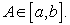The technique of determining the value of w(A) is called whitenization of A.

One usually defines w(A) = (1- t)a + tb, with t in [0, 1]. This is known as equal weight whitenization. When the distribution of A is unknown, we take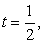which gives that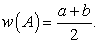### 4. The Assesment Method

The assessment method presented in this section was initially developed in 16.

Let G be a group of n objects participating in a certain activity. Assume that one wants to assess the mean performance of G in terms of the fuzzy linguistic expressions (grades) A = Excellent, B = Very good, C = Good, D = Fair and F = Unsatisfactory (Failed).

We introduce a numerical scale of scores from 0 to 100 and we correspond these scores to the linguistic grades as follows: A (100-85), B(84-75), C (74-60), D(59-50) and F(49-0). This correspondence, although it satisfies the common sense, it is not unique, depending on the user’s personal goals. For example, in a more strict assessment one could take A (100-90), B(89-80), C (79-70), D(69-60) and F(59-0), etc.

It is possible now to represent each linguistic grade by a GN, denoted for simplicity with the same letter. Namely, we introduce the GNs: A∈[85, 100], B∈[75, 84], C∈[60, 74], D∈[50, 59] and F∈[0, 49].

Let nA, nB, nC, nD and nF denote the numbers of the objects of G whose performance was characterized by the grades A, B, C, D and F respectively. Assigning to each object of G the corresponding GN we define the mean value of all those GNs to be the GN: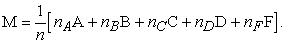It is logical now to consider the GN M as the representative of the group’s mean performance. Since the distributions of the GNs A, B, C, D and F are unknown, the same happens with the distribution of M. Therefore, if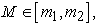we can take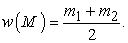It becomes evident that the value of w(M) determines the linguistic grade characterizing the group’s mean performance.

Remark: In earlier works (e.g. see 12, 13) he have assessed the mean performance of G by using the TFNs A = (85, 92.5, 100}, B = (75, 79.5, 84), C = (60, 67, 74], D = (50, 54.5, 59] and F = (0, 24.5 49] instead of the corresponding GNs used here. Assigning to each object of G the TFN corresponding to its performance we calculated the mean value M (a, b, c) of all those TFNs and we proved that the representative real value defuzzifying M is equal to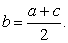Consequently the two assessment methods (TFNs and GNs) are equivalent to each other.

### 5. A Classroom Application

In the Calculus courses the emphasis is usually given on analytic representations and less effort is spent on presenting the concepts graphically. However, it has been reported that most students have no deep conceptual understanding of the derivative’s graphical representation and its correlation to its analytic definition 1, 3, 17.

The following classroom application took place recently at the Graduate Technological Educational Institute of Western Greece in the city of Patras with subjects the students of two different Engineering Departments of the School of Technological Applications. The instructor, who was the same for the two Departments, in order to help students to have a better understanding of the graphical representation of the derivative gave emphasis to the following points during the teaching procedure:

1. Connecting two points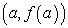and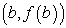on a given curve y = f(x) to construct its corresponding chord.

2. Calculating the slope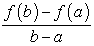of a secant line at a point as the other point b approaches it.

3. Defining the tangent line at a pointof the graph of a function y = f(x) and calculating its slope by the limit: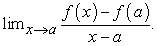4. Calculating the derivative f΄(a) at a point a from a given table of suitable values of the function y = f(x) in terms of the above process without using limits.

5. Presenting examples of constructing the graph of the derivative function f΄(x) when the graph but not the analytic formula of y = f(x) is given.

Next, a written test was given to the students of the two Departments involving the following questions:

Question 1: Use the values of the continuous function y = f(x) given below to approximate the value of its derivative at x = 2.

Calculating the slopes of the secant lines of the graph of y = f(x) defined by the point (2, 4) and the points οf Table 1 one finds that f ΄(2) = 4.

Question 2: Use the given graph of f to estimate the value of f΄(2)

• Figure 1. The graph of Question 2

One follows the same process with Question 1 calculating the coordinates of the corresponding points from the graph of Figure 1.

Question 3: Find the points and explain the reasons for which the function f in the below graph is not differentiable.

• Figure 2. The graph of Question 3

Although most students of both Departments were aware of the points where the function was not differentiable (x = -4 and x = 0), many of them did not give any explanations.

Question 4: The graph of a function y = f(x) is presented in the below figure. Observing that its tangent at the point (a, f(a)) is horizontal and its tangent at (b, f(b)) is vertical with respect to the x-axis, sketch the graph of the derivative function f΄(x).

• Figure 3. Graph of y = f(x) in Question 4

Since the tangent of the given graph at (a, f(a)) is parallel to the x-axis, its slope is equal to zero, which means that f ΄(α) = 0. Consequently, the graph of f ΄(x) intersects the x-axis at a. Also, from Figure 3 one observes that f(x) is strictly decreasing in the interval (-, a), which means that f ΄(x)<0, for all x in (-, a). Therefore, the graph of f ΄(x) in (-, a) lies under the x-axis. Further, the concavity of f(x) in (-, a), is upwards, which means that f ΄΄(x)>0. Consequently, the derivative function f΄(x) is strictly increasing in (-, a).

Working in the same way for the other intervals one obtains the draft design of the graph of f ΄(x) presented in Figure 4.

• Figure 4. The graph of f΄(x) in Question 4

The above questions are included in an unpublished work on teaching the derivatives done jointly by the present author, V. Borji, H. Alamolhodaei and F. Radmehr.

The results of the test are depicted in Table 2:

Assigning to each student the corresponding GN we calculate the mean values M1 and M2 of all those GNs for the Departments D1 and D2 respectively, which are approximately equal to: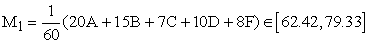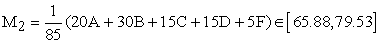Therefore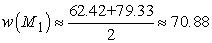and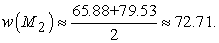Consequently both Departments demonstrated a good (C) mean performance, with the performance of the second Department being better.

In concluding, the emphasis given by the instructor during the teaching procedure to the points 1-5 presented in the beginning of this section help the students of both Departments to have a better understanding of the graphical representation of the derivative.

Remark: Consider the two extreme cases, where the maximal or the minimal possible numerical score corresponds to each student for each linguistic grade, e.g. for the first Department the 20 scores corresponding to A, are 100 or 85 respectively, etc. Then, the mean values of the student scores for the first Department are 79.93 and 62.42 respectively, while the value of w(M) is equal to the mean value of these two values. It is easy to check that this result is always true. Consequently, the assessment method with the GNs developed in this paper gives a good approximation of a group’s mean performance and therefore it is useful when no numerical scores are used and the group’s performance is assessed by qualitative grades.

### 6. Conclusion

In the paper at hand a method was developed with the help of GNs for the assessment of a group’s mean performance, which is useful when qualitative assessment grades and not numerical scores are used for this purpose. Although this method was proved to be equivalent with an analogous method using TFNs developed in earlier works, the required computational burden was significantly reduced. A classroom application was also presented on the graphical understanding of the derivatives illustrating our results.

GNs play in general an important role in science, engineering and in the everyday life for handling approximate data. In 18, for example, the eigenvalue problem of Correspondence Analysis with grey data was studied. The development of further applications of GNs to real life problems is one of the main plans of our future research.

### References

  Asiala, M., Cottrill, J., Dubinsky, E. & Schwingendorf, K.E. (1997), “The development of students’ graphical understanding of the derivative”, Journal of Mathematical Behavior, 16, 399-431. In article View Article  Clark J.M., Cordero, F., Cottrill, J., Czarnocha, B., DeVries, D.J., St. John, D., Tolias T.& Vidakovic, D. (1997), “Constructing a schema: The case of the chain rule”, Journal of Mathematical Behavior, 16, 345-364. In article View Article  Ferrini–Mundy, J. & Graham, K. (1994), “Research in calculus learning: Understanding limits, derivatives, and integrals”, in E. Dubinsky & J. Kaput (Eds.), Research issues in undergraduate mathematics learning, pp. 19-26, Mathematical Association of America. In article  Orton, A. (1983), “Students’ Understanding of Differentiation”, Educational Studies in Mathematics, 14, 235-250. In article View Article  Tall, D. (1993), “Students’ Difficulties in Calculus”, Plenary Address, Proceedings of ICME-7, 13-28, Québec, Canada. In article View Article  Thompson, P.W. (1994), “Images of rate and operational understanding of the fundamental theorem of calculus”, Educational Studies in Mathematics, 26, 229-274. In article View Article  Maharaj, A. (2005), Investigating the Senior Certificate Mathematics examination in South Africa: Implications for teaching, Ph.D. thesis, Pretoria, University of South Africa. In article View Article  Andresen, M. (2007), “Introduction of a new construct: The conceptual tool “Flexibility””, The Montana Mathematics Enthusiast, 4, 230-250. In article View Article  Hähkiöniemi, M. (2004), “Perceptual and symbolic representations as a starting point of the acquisition of the derivative”, Proceedings of the 28th Conference of the International Group for the Psychology of Mathematics Education, 3, 73-80. In article View Article  Maharaj, A. (2010), “An APOS Analysis of Students’ Understanding of the Concept of a Limit of a Function”, Pythagoras, 71, 41-52. In article View Article  Roorda, G., Vos, P. & Goedhart, M. (2009), “Derivatives and applications; development of one student’s understanding”, Proceedings of CERME 6, Available at www.inrp.fr/editions/cerme6. In article View Article  Voskoglou, M. Gr. (2017), Finite Markov Chain and Fuzzy Logic Assessment Models; Emerging Research and Opportunities, Createspace.com. – Amazon, Columbia, SC, USA. In article  Voskoglou, M. Gr. (2015), “Defuzzification of fuzzy numbers for student assessment”, American Journal of Applied Mathematics and Statistics, 3(5), 206-210. In article View Article  Liu, S. F. & Lin, Y. (Eds.) (2010), Advances in Grey System Research, Springer, Berlin – Heidelberg. In article View Article  Moore, R.A., Kearfort, R. B. & Clood, M.J. (1995), Introduction to Interval Analysis, 2nd Printing, SIAM, Philadelphia. In article View Article  Voskoglou, M. Gr. & Theodorou, Y. (2017), “Application of Grey Numbers to Assessment Processes”, International Journal of Applications of Fuzzy Sets and Artificial Intelligence, 7, 59-72. In article  Zandieh, M.J. (2000), “A Theoretical Framework for Analyzing Student Understanding of the Concept of Derivative”, CBMS Issues in Mathematics Education, 8, 103-122. In article View Article  PubMed  Alevizos, P. D., Theodorou, Y. & Vrahatis, M.N. (2017), “Correspondence Analysis with Grey Data: The Grey Eigen Value Problem”, The Journal of Grey System, 29(1), 92-105. In article View ArticleThis work is licensed under a Creative Commons Attribution 4.0 International License. To view a copy of this license, visit http://creativecommons.org/licenses/by/4.0/